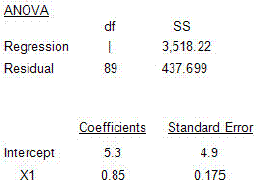### CFA Practice Question

There are 208 practice questions for this study session.

### CFA Practice Question

In comparing the relation of company sales with GDP, your regression software provides the following output:Is it possible that the regression coefficient is insignificant at the 95% level of confidence?
A. No since the t-statistic of 4.86 greatly exceeds the critical values of the insignificance acceptance range.
B. No, since the t-statistic is within the critical values of the insignificance acceptance range.
C. Yes, since the t-statistic is not large enough to be classified as insignificant.
Explanation: t-start = 0.85/0.175 = 4.86.

t-critical at df = 89 and α = 0.025 is 2.0.

Note that with a large sample size (over 30), we can begin to rely on the more familiar z-statistics.